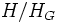# Nilpotent quotient-by-core subgroup

This article defines a subgroup property: a property that can be evaluated to true/false given a group and a subgroup thereof, invariant under subgroup equivalence. View a complete list of subgroup properties[SHOW MORE]
This is a variation of normality|Find other variations of normality | Read a survey article on varying normality

## Contents

BEWARE! This term is nonstandard and is being used locally within the wiki. [SHOW MORE]

## Definition

### Symbol-free definition

A subgroup of a group is said to be nilpotent quotient-by-core if the quotient-by-core of this subgroup (that is, its quotient by its normal core) is a nilpotent group.

### Definition with symbols

A subgroup$H$ in a group$G$ is termed nilpotent quotient-by-core if$H/H_G$ is a nilpotent group where$H_G$ denotes the normal core of$H$ (or the intersection of conjugates of$H$).